### Approximate Methods: Virtual Work Method

The statement of the equilibrium equations applied to a set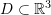is as follows. Assuming that at equilibrium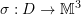is the symmetric Cauchy stress distribution on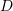and that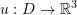is the displacement vector distribution and knowing the relationship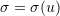, then the equilibrium equation seeks to find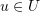such that the associatedsatisfies the equation: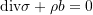where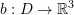is the body forces vector distribution on,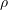is the mass density, and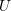is the space of all possible displacement functions applied to, i.e.,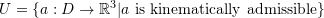. The term “Kinematically admissible” inindicates that the space of possible solutions must satisfy the boundary conditions imposed on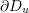(as stated below) and any differentiability constraints.

The boundary conditions for the equations of equilibrium are usually given on two parts of the boundary ofdenoted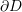. On the first part,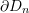, the external traction vectors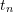are known so we have the boundary conditions forsince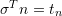(is the normal vector to the boundary). On the second part,, the displacement is given.

Alternatively, the statement of the principle of virtual work states that at equilibrium,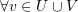where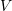is the space of at least once differentiable vector functions on, i.e.,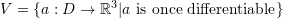: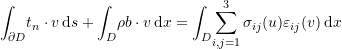where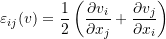As shown in the principle of virtual work section, one of its major applications is to find approximate solutions for the equations of equilibrium. This can be obtained by assuming that the solution u has a particular form with a finite number of unknowns, i.e., by looking forin a subset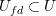that is finite dimensional but still able to approximate functions in. The example in the principle of virtual work section utilizes polynomial approximations to find an approximate solution for the displacement field. The finite element method in the next sections utilizes piecewise affine functions to approximate the displacements. In this case the setis expanded to allow for possible displacement functions that are not differentiable across element boundaries: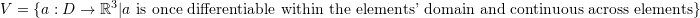.

### Problems

Solve the problems in the Rayleigh Ritz method section using the Virtual Work Method.